# Panex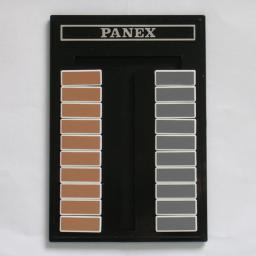Panex is a puzzle that was invented by Toshio Akanuma. It resembles the tower of Hanoi, but it is in fact a lot more difficult. It has two towers of 10 pieces. Instead of being made from disks threaded on pegs, the towers are made of pieces that slide along a track. There are 3 vertical sections of track, similar to Hanoi's three pegs, where a tower can be built. These 'pegs' are exactly long enough to hold a completed tower plus one extra piece. A horizontal track connects the top of the three pegs, so that pieces can move from the very top of one 'peg' to the next. At the start, the left peg contains a blue tower, the right peg an orange tower, and the middle peg is empty. The aim is to swap the two towers. The problem is however that disks cannot freely slide all the way down each peg. A disk cannot move to a position lower than where it belongs in a finished tower.

There are two versions of the Panex puzzle. Panex Silver has blue and orange towers as explained above. From the size of blue/orange shape on each piece you can immediately see at which level it belongs in the finished tower. The Panex Gold however has pieces that look identical, one tower grey and the other brown, making it more confusing still.

This is one of the most difficult puzzles on my site. Partly this is because it is hard to do, but also because the solution is extremely long. It is thought that the optimal solution of Panex needs more than 31000 moves. The Japanese collector whom I bought it from did solve it (the Silver version), but it took him 2 months, an hour a day.

If your browser supports JavaScript, then you can play the Panex by clicking the link below. Its built-in solver is not optimal, but is pretty good considering that it can solve any random position.

## Analysis:

Virtually all of the analysis below is based on the paper by Mark Manasse, Danny Sleator, and Victor K. Wei which can be downloaded from Nick Baxter's Panex page.

Recursion

Like the towers of Hanoi, this puzzle can be solved recursively. One basic task is swapping a tower of n disks on a side peg with a single disk at level n on the middle peg. The picture below shows this. Let's call this task Sn, a swap of level n.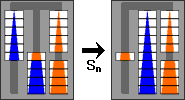A simpler related task is to move a tower of n disks from a side peg to the middle peg. This similar to the swap Sn above, except that one piece is missing. If we can do Sn, then we can certainly do this by just leaving out any moves that would involve the missing piece. Let's call this task Tn, a transfer of level n.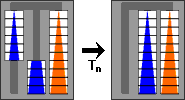We can now set up the recursion, defining these level n tasks using smaller tasks of level n-1. The sequence of pictures below shows how this is done.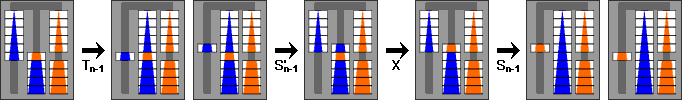Sn = Tn-1 + S'n-1 + X + Sn-1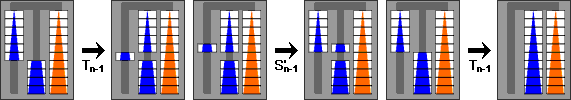Tn = Tn-1 + S'n-1 + Tn-1

Here the inverse of a move or move sequence is denoted by a tick mark. Also new is the move sequence denoted by X, which consists of 4 moves and swaps the top two disks on the middle peg. Note how Tn is just like Sn but without the unnecessary X swap.

The cases T1 and S1 are trivial (1 and 3 moves respectively), so this shows that we can definitely perform all these swaps and transfers for any number of disks. To actually solve the puzzle we need to swap the two towers on the outside pegs. We can swap the two bottom disks as follows: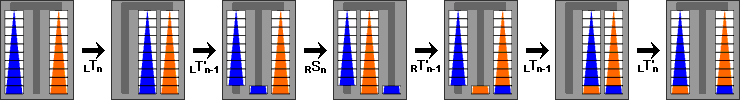Xn = LTn + LT'n-1 + RSn + RT'n-1 + LTn-1 + LT'n

The L and R subscripts indicate on which side the move sequence is performed.
We can repeat this exchange for each level of the puzzle: X10 + X9 + X8 + ... + X1 exchanges the pieces at each level, thus exchanging the towers and solving the puzzle. This at least proves the puzzle can be solved.

Improvement

The method above is not at all optimal. First of all, the recursive definition of Tn has many cancellations in it:

Tn = Tn-1 + S'n-1 + Tn-1
= Tn-1 + ( Tn-2 + S'n-2 + X + Sn-2 )' + ( Tn-2 + S'n-2 + Tn-2 )
= Tn-1 + S'n-2 + X + Sn-2 + T'n-2 + Tn-2 + S'n-2 + Tn-2
= Tn-1 + S'n-2 + X + Tn-2

Let's now see how many moves this would take, assuming that there are no cancellations or simplifications anywhere. Let tn, sn, and xn be the number of moves of Tn, Sn and Xn respectively. From the relations above we get the recurrence relations:

sn = 4 + tn-1 + 2sn-1
tn = 4 + tn-1 + tn-2 + sn-2
xn = 3tn-1 + 2tn + sn

If we use the boundary conditions s1=3, t1=1, we get the following table:

nSnTnXnSolution
13155
21152429
3311372101
47933184285
519581456741
647519711121853
7115147726964549
827831153652011069
9672327851575226821
10    16235    6725    38040    64861

For ten disks this gives 64861 moves. Fortunately this is not optimal, so the real number is less. In particular, T2 and S2 need not be done in 5 and 11 moves respectively, but can easily be done in 3 and 8 moves, because we can make more efficient use of the space in the top row of the puzzle. Similarly, T3 can be done in 9 moves. This results in the following table:

nSnTnXnSolution
13155
2831722
32395072
45924134206
514660338544
63561478301374
786335720183392
8208786448868278
9504220881181020088
10    12176    5043    28526    48614

It turns out that there are some small move cancellations here or there in S and T, but even optimally T10 needs 4875 moves so this is a rather good method for it. On the other hand, there is a much better way of exchanging the towers than doing the pairs at each level from the bottom up, because we can do much of the work on the top pieces during the exchange of the bottom pair. This is easiest to explain using the following sequence of moves, which we will call Zn: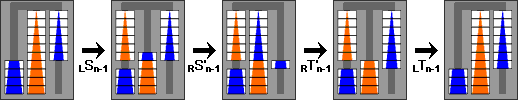Zn = LSn-1 + RS'n-1 + RT'n-1 + LTn-1

This transfers the top piece of the left stack across to the bottom of the right stack.

The two towers can now be exchanged as follows: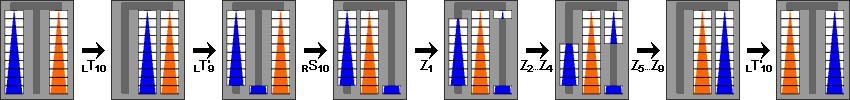Solution10 = LT10 + LT'9 + RS10 + Z1 + Z2 + Z5 + ... + Z8 + Z9 + LT'10

The last Z9 and LT'10 can provide some more cancellation:
Z9 + LT'10 = ( LS8 + RS'8 + RT'8 + LT8) + (LT9 + LS'8 + X + LT8)'
= LS8 + RS'8 + RT'8 + LT8 + LT'8 + X + LS8 + LT'9
= LS8 + RS'8 + RT'8 + X + LS8 + LT'9

The idea behind this solution is that as soon as you have the largest blue piece in its correct place, you first fix the remaining blue ones above it. It is easier to do that first, separating the colours, because the orange tower can be built afterwards in its correct place without disturbing the blue pieces.

Given also that Z1 is one move, and Z2 seven, this leads to the following table:

nSnTnZn    Sum Zn    Solution
13111
2837816
3239223050
459246494140
514660166260366
6356147412672922
7863357100616782276
82087864244041185556
95042208859021002013486
10    12176    5043    14260    24280    32642

This improves on the previous method by more than 30%. The best known solution uses this method, shortened slightly by some more simplifications and cancellations. It still uses 31537 moves, and that solution method has been proved optimal by computer search.

Solving any position

Given that the solution is so long, it is quite likely that at some point you will lose track of where you were in the solution. It is possible to deduce what to do by examining where the largest pieces are, and then the next largest pieces, and so on. Of course, this assumes the pieces are distinguishable (i.e. the Panex Silver, not Gold). To do this, you must of course understand what route these pieces take during the solution. Let's first look at the largest blue piece.

1. The large blue piece starts at the bottom of the left column.
2. All the pieces above it on the left peg are removed (and piled up on the middle and/or right pegs).
3. The large blue piece is moved to the middle peg, and slowly works its way down through the pieces of the middle peg, all the way until it is at the bottom.
4. Now the largest other piece on the middle or right peg is freed up, and worked down the middle peg, until it is the only piece on the middle peg other than the large blue piece.
5. The large blue piece swaps with that other piece, rising upwards a bit.
6. Steps d and e are repeated with the second largest piece that can be found on the middle or right peg, then the next largest, and so on. The blue piece therefore bubbles upwards on the middle peg while all the other pieces from the right peg build up a tower underneath it.
7. The blue piece then can be moved onto the empty right peg, to its solved position.